How to Learn in 24 Hours?The Rapid Learning Movie

 Need Help? M-F: 9am-5pm(PST): Toll-Free: (877) RAPID-10 US Direct: (714) 692-2900 Int'l: 001-714-692-2900 24/7 Online Technical Support: The Rapid Support Center Secure Online Order:Need Proof? Testimonials by Our Users

 Rapid Learning Courses: MCAT in 24 Hours (2015-16) USMLE in 24 Hours (Boards) Chemistry in 24 Hours Biology in 24 Hours Physics in 24 Hours Mathematics in 24 Hours Psychology in 24 Hours SAT in 24 Hours ACT in 24 Hours AP in 24 Hours CLEP in 24 Hours DAT in 24 Hours (Dental) OAT in 24 Hours (Optometry) PCAT in 24 Hours (Pharmacy) Nursing Entrance Exams Certification in 24 Hours eBook - Survival Kits Audiobooks (MP3)

 Tell-A-Friend: Have friends taking science and math courses too? Tell them about our rapid learning system.Home »  Mathematics »  High School Algebra 2

Logarithmic Functions

 Topic Review on "Title": Logarithmic function: The inverse function of the exponential function is called the logarithmic function and is denoted as: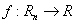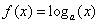where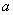is the base of the logarithm andProperties of logarithmic functions: 1) If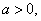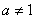and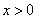then. 2) Ifthen. 3) If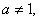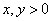then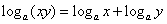. 4) Ifthen. 5) Ifand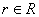then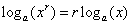.

Rapid Study Kit for "Title":
 Flash Movie Flash Game Flash Card Core Concept Tutorial Problem Solving Drill Review Cheat Sheet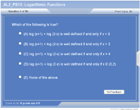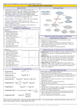"Title" Tutorial Summary : Logarithms and how they relate to exponentials are described in this tutorial.  The graphs of logarithms follow the same rules as the ones of exponentials except they are not defined at zero. The concept of inverse functions is utilized to confirm that logarithms have inverses. Examples of logarithms and their graphs are shown in the example problems. Logarithms are not defined at 0 compared to exponentials. Logarithms are solved using their properties involving them and are presented in the examples.

 Tutorial Features: Specific Tutorial Features: • Examples to illustrate the properties and applications of logarithms. • Animated diagrams to actually show the relationship between an exponential and a logarithm with the use of the inverse function principle. • Problem-solving techniques are used to work out and illustrate the example problems, step by step. Series Features: • Concept map showing inter-connections of new concepts in this tutorial and those previously introduced. • Definition slides introduce terms as they are needed. • Visual representation of concepts • Animated examples—worked out step by step • A concise summary is given at the conclusion of the tutorial.

 "Title" Topic List: Logarithms functions and their properties Definition of logarithms Properties of logarithms Logarithmic equationsCommon and Natural Logarithms Euler Number Logarithms with different bases Application of logarithms

See all 24 lessons in High School Algebra 2, including concept tutorials, problem drills and cheat sheets:
Teach Yourself High School Algebra 2 Visually in 24 Hours